Disclosure: This post may contain affiliate links, meaning when you click the links and make a purchase, we receive a commission.

# Exponential Notation in Excel & How to Turn Off Auto Scientific Notation!

This article will at first introduce you to the scientific number system and number precision definition. Then you will know about the highest and lowest number limitations in Excel. At the end of the article, I have narrated how you can turn off auto scientific notation in Excel.

Disclaimer: “Turn off scientific notation in Excel” – with this phrase I actually don’t mean I am going to TURN OFF the scientific notation in Excel. I am actually changing the way the numbers are displayed in Excel cells. (Thanks Mark and Helen for pointing this out)

Let’s start today’s post thinking about a few questions. How big a number can Excel handle? Or how many types?  Or with what precision can it report statistics to its users.

Read More: TEXT and FORMAT Functions in Excel [68 Examples]

First, we will discuss a few examples of these situations:

## How Scientific Notation Works

Sometimes, especially while using a calculator, you may end up with a very long number. For example, you have a very large number like this: 1234567894578215153456789, this number has 25 digits. Or you may face a small number like this: 0.12345621345722156652231

To use these types of numbers conveniently, you can express them in scientific notation.

Let’s take a smaller number; in scientific notation, 7245 becomes 7.245E+3;

How? The decimal point moved 3 digits left. So, the scientific notation is 7.245E+3, +3 as the decimal point has moved left.

So, you will express the movement with an E.

In scientific notation 183857.419 becomes 1.83857419E+5 as for this number, the decimal point has moved 5 digits left.

In scientific notation, this small number, 0.00007245 becomes 7.245E-5. As the decimal point has moved 5 digits right. In the same way, number 0.0000000625431 will become 6.25431E-8, as the decimal point has moved 8 digits right.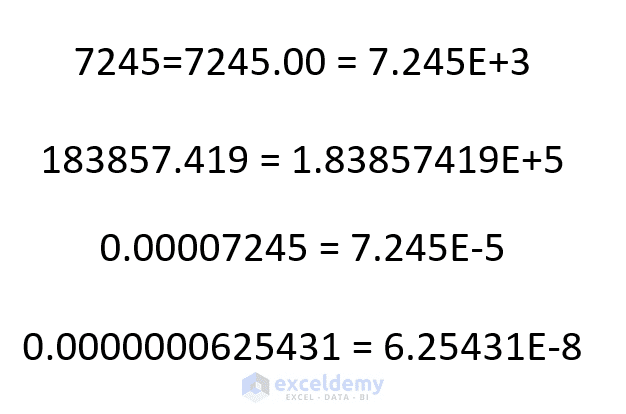## What is Number Precision?

Now let’s discuss number precision. The precision of a number is how many digits of a number are shown.

Considering the same numbers 7.245E+3 number’s precision is 4 as it is showing that many digits.

1.83857419E+5 number’s precision is 9; as it’s showing 9 digits.

7.245E-5 number’s precision is 4 as it has 4 digits.

And lastly 6.25431E-8 number’s precision is 6 as it is showing that many digits.

## What can Excel handle

### #Highest and Lowest Number Limits in Excel 2013 & 2016

The largest positive number that you can store in a worksheet cell is 9.9E+307.

It is 99 then three hundred and six zeros. It is an extraordinarily large number, of course.

The smallest negative number you can store in a worksheet cell is -9.9E-307. It is minus zero point three hundred and six zeros, and then 99.

### #Numbr Precision in Excel

Excel’s numbers are precise up to 15 digits.

If you enter a number that has more than 15 digits, the digits after 15 will be converted to Zeros. For example, enter number 1234, 56789, 1234, 56789 in a worksheet cell and look at the Formula bar (don’t hit enter yet).

There are 18 digits of this number.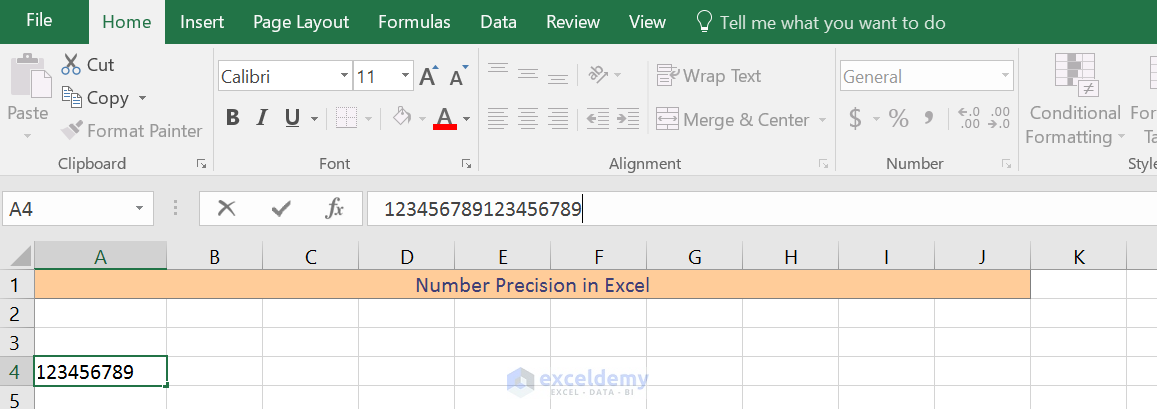When I hit enter, the number gets converted to scientific notation. You can see how Excel understood it by looking at the formula bar. There are 15 digits intact but the last 3 digits are converted to zeros.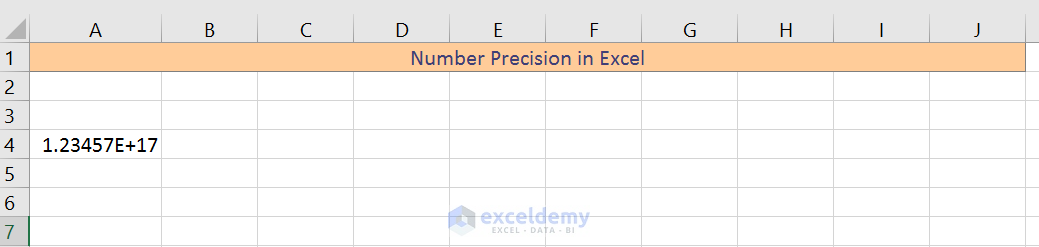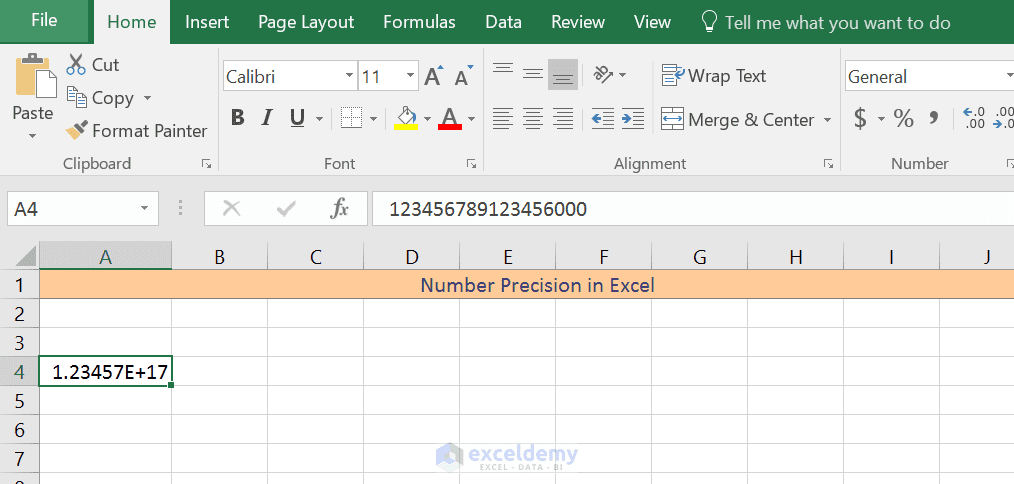Here is another number 20 digits long this time.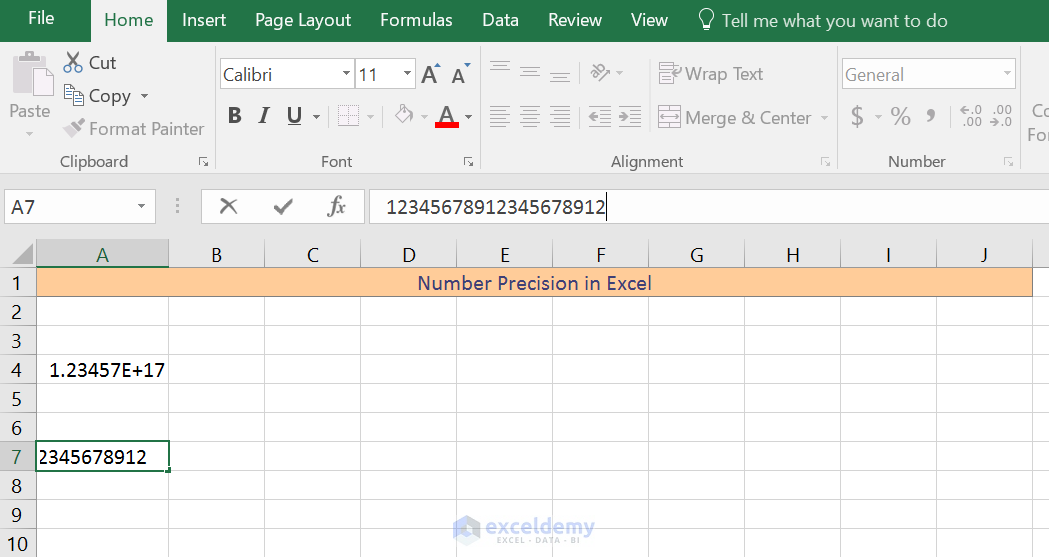Press Enter.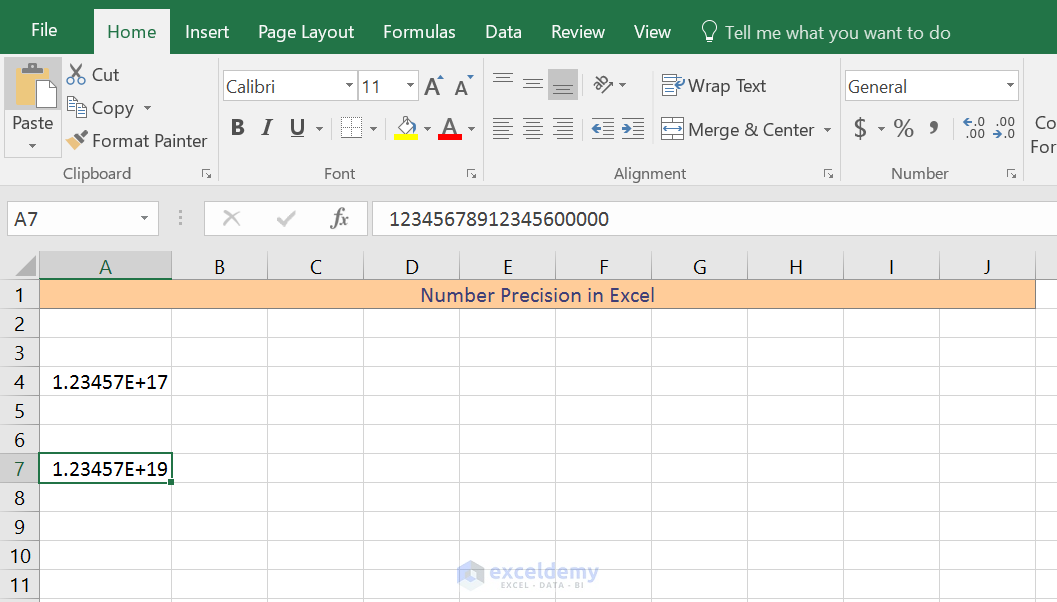To see how the number is saved, select the cell. You see, the first 15 digits are intact again and the rest 5 digits are transferred to zeros.

So, if you save a number that has more than 15 digits, then remember that the result based on this number will not be as accurate as you may expect. This precision may seem quite limiting, but in practice, it rarely causes any problem.

## Turn off scientific notation in Excel

So, Excel automatically turns a number in scientific notation if the cell width is not sufficient for the number.

Sometimes, you might want to stop this auto converting of numbers into scientific notation.

How can you do that?

Simply select the cell where the scientifically noted number sits. And format the cell with Number format.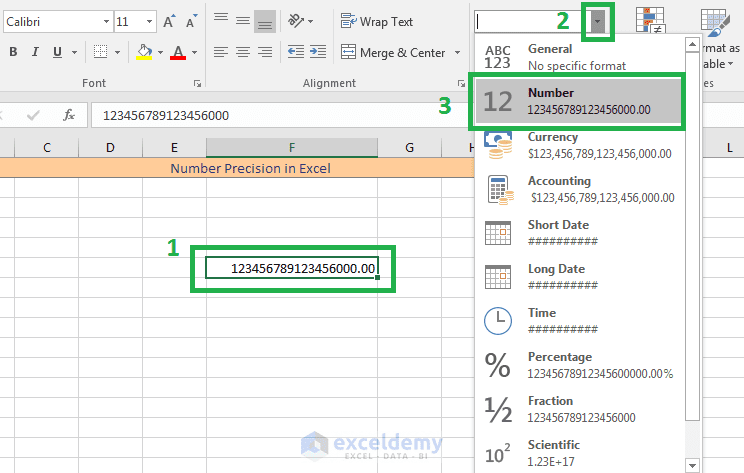If you format a cell with the Number format from the Number format drop-down, then by default you will get two places after the decimal point.

To avoid the decimal places, you can use the Format Cells dialog box. Select Number option from the left window, and on the right window, make the decimal places to zero.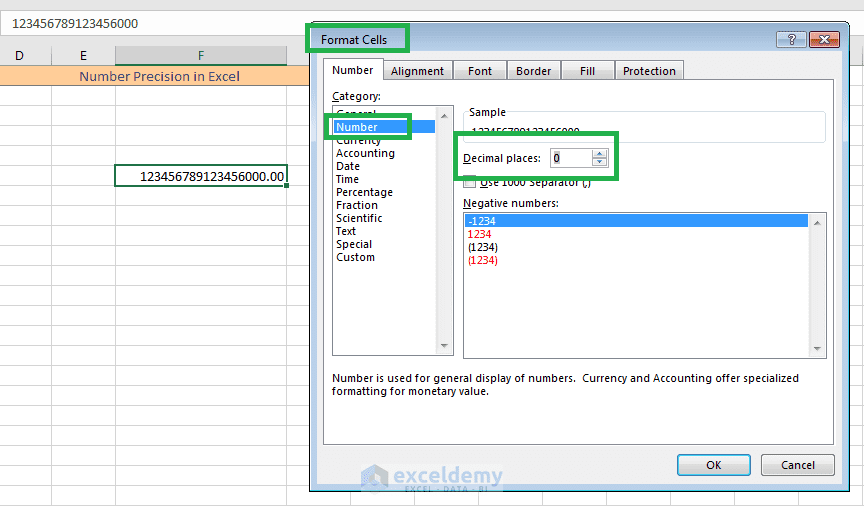So, this was number theory folks, stay tuned for some more Excellence.

Scientific-Number-System-Number-Precision.xlsx

Hello!
Welcome to my Excel blog! It took me some time to be a fan of Excel. But now I am a die-hard fan of MS Excel. I learn new ways of doing things with Excel and share here. Not only how to guide on Excel, but you will get also topics on Finance, Statistics, Data Analysis, and BI. Stay tuned!

1. ReplyMatt D. Dec 25, 2017 at 12:11 AM

Hi, I work with UPC codes, and Excel has this habit of converting them into scientific notation. It’s super annoying to say the least. This solution is just as others have provided, it stops that instance from happening but does not turn this “feature” off. How can I accomplish this?

Thank you.

Matt

2. ReplyMar 21, 2018 at 7:38 PM

“Turn off scientific notation in Excel”

You are not turning it off, you change the way the number is displayed, which isnt the same thing.
You might want to change that statement, because it is misleading.

Thank you,
Mark

• ReplySep 1, 2018 at 2:17 PM

Thanks, Mark.

3. ReplyHelen Aug 31, 2018 at 4:44 AM

I am disappointed at the click-bait on this site. As Mark mentioned, you are not turning off the scientific notation, and this does nothing to display the actual number unless you only have two decimal places in your number. Given that you specifically point out that this is a problem with small numbers, it seems especially unhelpful to just pretend that this solved the problem.

• ReplySep 1, 2018 at 2:17 PM

Hi Helen,
Thanks for the feedback.
And I will work with the click-baits to make it more user-friendly.
Thanks and regards